Next: Running the test Up: Boundary condition example Previous: Initial conditions   Contents

### Boundary conditions

Eastern Boundary: open
The eastern boundary fluxes normal to the faces are specified while values used for advection of momentum and scalars are upwinded
Boundary fluxes
As specified in boundaries.c, the eastern boundary is an open boundary and employs the linearized boundary condition on the velocity with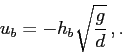where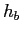is the free-surface height,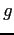is gravitational acceleration, and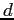is the depth, and the minus sign implies flux out of the domain when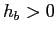. This is enforced in the OpenBoundaryFluxes function with the lines
if(grid->yv[ib]>50) {
for(k=grid->etop[j];k<grid->Nke[j];k++)
ub[j][k]=-phys->h[ib]*sqrt(prop->grav/grid->dv[ib]);
...

The ib index is the index of the cell adjacent to the boundary, and the if-statement is required to distinguish this eastern outflow boundary from the southern boundary. Note that because the edge lengths are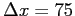m, this implies that the distance between the Voronoi points and the boundary edges is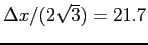m. Therefore, we know that if the location of the boundary Voronoi point ib adjacent to a boundary edge j is greater than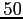m, it must be the eastern boundary.
Boundary velocities
At the eastern open boundary, the boundary velocities which are used for advection of momentum out of the domain are given by the upwind velocity components. This is specified in the BoundaryVelocities function with the lines
if(grid->yv[ib]>50) {
for(k=grid->etop[j];k<grid->Nke[j];k++) {
phys->boundary_u[jptr-grid->edgedist][k]=
phys->uc[ib][k];
phys->boundary_v[jptr-grid->edgedist][k]=
phys->vc[ib][k];
phys->boundary_w[jptr-grid->edgedist][k]=
0.5*(phys->w[ib][k]+phys->w[ib][k+1]);
}

Because the flux of momentum is calculated at the vertical centers of the vertical faces, the upwind vertical velocity is interpolated from the upper (k) and lower (k+1) faces of the kth cell.
Boundary scalars
The outflow conditions on the scalars are imposed by specified the upwind scalar quantity at the outflow face. This is set in the function BoundaryScalars with the lines
for(k=grid->ctop[ib];k<grid->Nk[ib];k++) {
phys->boundary_T[jptr-grid->edgedist][k]=phys->T[ib][k];
phys->boundary_s[jptr-grid->edgedist][k]=phys->s[ib][k];
}


Southern boundary: specified
All quantities at the southern boundary are specified. Since this example simulates a river inflow, the velocity and scalar fields are specified along only part of the southern boundary, while the rest of the boundary is kept at zero inflow to represent a solid boundary. This requires if-statements to determine which of the southern boundary edges fall between the extent of the inflowing river. An alternative is to set the markers of the southern boundary edges that fall within the boundaries of the river to 2, and the rest to 1.
Boundary fluxes Because the velocity components at the southern boundary are specified, the incoming boundary flux is computed in OpenBoundaryFluxes using the velocity components specified in the BoundaryVelocities function, but only within the extent of the 300 m wide river, which is defined for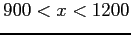m (4 cell faces), as in:
if(grid->xv[ib]>900&&grid->xv[ib]<1200)
for(k=grid->etop[j];k<grid->Nke[j];k++)
ub[j][k]=
phys->boundary_u[jptr-grid->edgedist][k]*grid->n1[j]+
phys->boundary_v[jptr-grid->edgedist][k]*grid->n2[j];

Since boundary_u and boundary_v store the inflow velocity components, then this is setting the flux at the inflow to the normal component of the velocity at the inflow, i.e.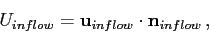where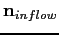is, by convention, the inward pointing normal at the boundary face.
Boundary velocities The north-south velocity is specified at the inflow forover a specified depth, which represents the river inflow, such that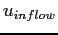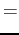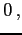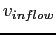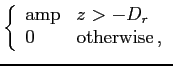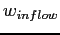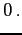where amp is specified in suntans.dat as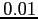m s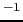, and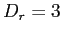m is the inflow depth. In boundaries.c, this inflow is implemented with
if(grid->xv[ib]>900 && grid->xv[ib]<1200) {
z=0;
for(k=grid->etop[j];k<grid->Nke[j];k++) {
z-=0.5*grid->dzz[ib][k];
if(z>-3.0)
phys->boundary_v[jptr-grid->edgedist][k]=prop->amp;
z-=0.5*grid->dzz[ib][k];
}
}

All other components are set to zero at the beginning of the main loop in the function BoundaryVelocities with
for(k=grid->etop[j];k<grid->Nke[j];k++) {
phys->boundary_u[jptr-grid->edgedist][k]=0;
phys->boundary_v[jptr-grid->edgedist][k]=0;
phys->boundary_w[jptr-grid->edgedist][k]=0;
}

Boundary scalars The inflow boundary condition for the salinity (or density, when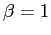) field represents an inflow of fresh water with a density anomaly of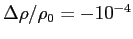, with a surface depth ofm, such that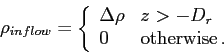The inflow boundary condition for temperature is a no-gradient condition, and as a result the temperature just outside the boundary is equal to that just inside the boundary. These boundary conditions for salinity (density) and temperature are given in boundaries.c as
if(grid->yv[ib]<50)
if(grid->xv[ib]>1000 && grid->xv[ib]<1200)
for(k=grid->ctop[ib];k<grid->Nk[ib];k++) {
z-=0.5*grid->dzz[ib][k];
if(z>-3.0) {
phys->boundary_T[jptr-grid->edgedist][k]=phys->T[ib][k];
phys->boundary_s[jptr-grid->edgedist][k]=-0.0001;
} else {
phys->boundary_T[jptr-grid->edgedist][k]=phys->T[ib][k];
phys->boundary_s[jptr-grid->edgedist][k]=0;
}
z-=0.5*grid->dzz[ib][k];
}Next: Running the test Up: Boundary condition example Previous: Initial conditions   Contents
2014-08-06